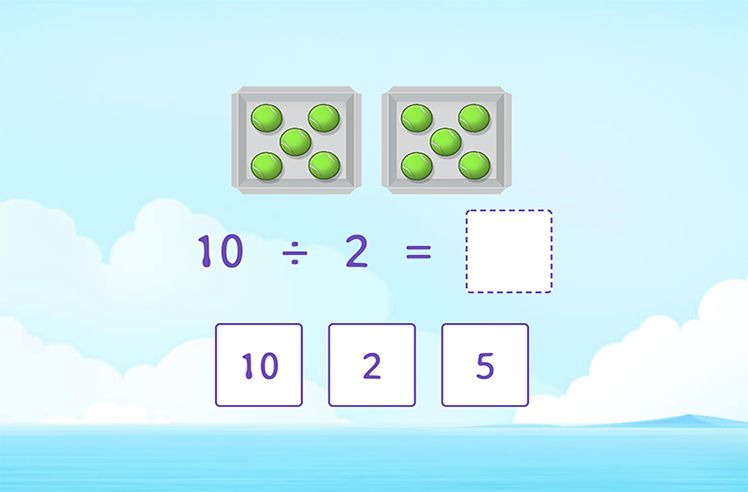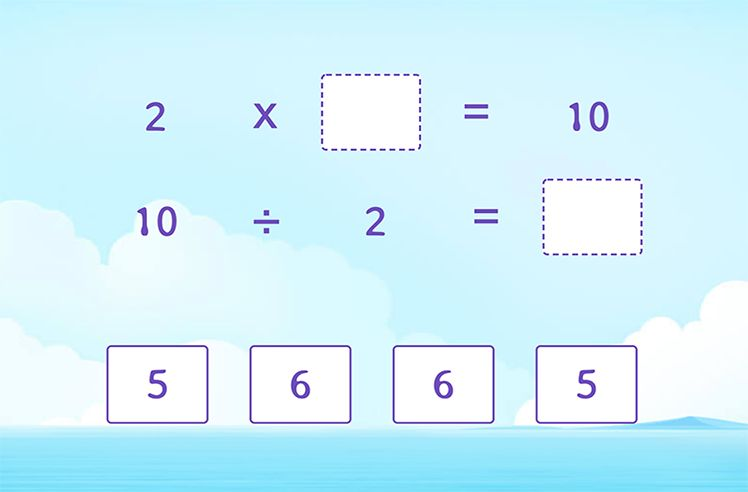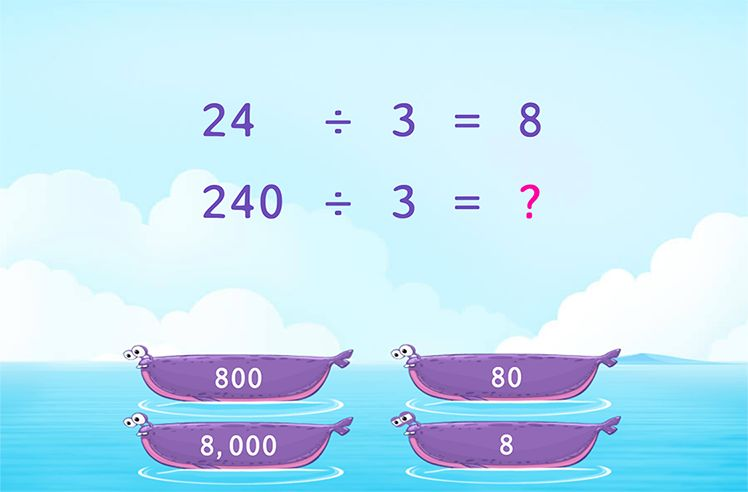# Remainder – Definition with Examples

## What is Remainder?

Remainder means something which is ‘left over’ or ‘remaining’.

If you have 9 toffees and you share it equally with your four friends. How many toffees will you have?

If you give two toffees each to your friends, you would have shared 8 toffees.  Only1 toffee will remain with you, and this leftover of 1 toffee is called the remainder.

Mathematically we can write the above expression as:

9 ÷ 4 = 2 remainder 1

Where 9 is the dividend, 4 is the divisor, 2 is the quotient, and 1 is the remainder.

On dividing 22 by 3.

We get 3 equal parts of 7, that add up to 21

3 x 7 = 21.

We are left with 1. This 1 is the remainder.

When one number cannot divide another number completely, it le get a remainder.

Examples:

## Properties of Remainder:

• When one number divides another number completely, the remainder is 0.
• The remainder is always less than the divisor. If the remainder is greater than the divisor, it means that the division is incomplete.
• It can be greater than or lesser than the quotient. For example; when 41 is divided by 7, the quotient is 5 and the remainder is 6. Here the remainder is greater than the quotient.Introduction to Division

Play NowDivision Facts

Play Now